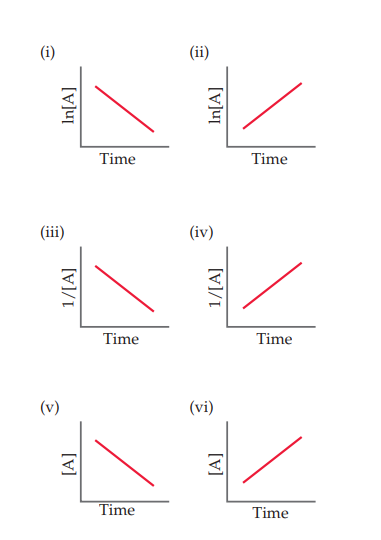×
Get Full Access to Chemistry: The Central Science - 14 Edition - Chapter 14 - Problem 14.8
Get Full Access to Chemistry: The Central Science - 14 Edition - Chapter 14 - Problem 14.8

×

# ?Which of the following linear plots do you expect for a reaction $$A \longrightarrow$$ products if the kinetics are (a) zero order,ISBN: 9780134414232 1274

## Solution for problem 14.8 Chapter 14

Chemistry: The Central Science | 14th Edition

• Textbook Solutions
• 2901 Step-by-step solutions solved by professors and subject experts
• Get 24/7 help from StudySoup virtual teaching assistantsChemistry: The Central Science | 14th Edition

4 5 1 395 Reviews
30
1
Problem 14.8

Which of the following linear plots do you expect for a reaction $$A \longrightarrow$$ products if the kinetics are

(a) zero order,

(b) first order, or

(c) second order? [Section 14.4]Text Transcription:

A \longrightarrow

Step-by-Step Solution:

Step 1 of 5) Plant nutrients, particularly nitrogen and phosphorus, contribute to water pollution by stimulating excessive growth of aquatic plants. The most visible results of excessive plant growth are floating algae and murky water. What is more significant, however, is that as plant growth becomes excessive, the amount of dead and decaying plant matter increases rapidly, a process called eutrophication (Figure 18.19). The processes by which plants decay consumes O2, and without sufficient oxygen, the water cannot sustain animal life. The most significant sources of nitrogen and phosphorus compounds in water are domestic sewage (phosphate-containing detergents and nitrogen-containing body wastes), runoff from agricultural land (fertilizers contain both nitrogen and phosphorus), and runoff from livestock areas (animal wastes contain nitrogen).Eutrophication. This rapid accumulation of dead and decaying plant matter in a body of water uses up the water’s oxygen supply, making the water unsuitable for aquatic animals.

Step 2 of 2

##### ISBN: 9780134414232

This full solution covers the following key subjects: . This expansive textbook survival guide covers 29 chapters, and 2820 solutions. The full step-by-step solution to problem: 14.8 from chapter: 14 was answered by , our top Chemistry solution expert on 10/03/18, 06:29PM. The answer to “?Which of the following linear plots do you expect for a reaction $$A \longrightarrow$$ products if the kinetics are (a) zero order, (b) first order, or (c) second order? [Section 14.4] Text Transcription:A \longrightarrow” is broken down into a number of easy to follow steps, and 34 words. Chemistry: The Central Science was written by and is associated to the ISBN: 9780134414232. This textbook survival guide was created for the textbook: Chemistry: The Central Science, edition: 14. Since the solution to 14.8 from 14 chapter was answered, more than 214 students have viewed the full step-by-step answer.

## Discover and learn what students are asking

Calculus: Early Transcendental Functions : Differential Equations: Separation of Variables
?In Exercises 1-14, find the general solution of the differential equation. $$\frac{d y}{d x}=\frac{6-x^{2}}{2 y^{3}}$$

Statistics: Informed Decisions Using Data : The Normal Approximation to the Binomial Probability Distribution
?In Problems 5–14, a discrete random variable is given. Assume the probability of the random variable will be approximated using the normal distributio

Unlock Textbook Solution

?Which of the following linear plots do you expect for a reaction $$A \longrightarrow$$ products if the kinetics are (a) zero order,